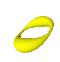Summer 2004

Mathematics Maths21a Summer 2004

Multivariable Calculus

Office: SciCtr 434
Email: knill@math.harvard.eduMathematica Laboratory

 Project gallery, Summer 2004

Availability Mathematica is installed on many computers in the basement of the Science center. It might also be installed on some computers in the houses. If you want to try an installation of mathematica on your own computer, get it here. Note that you have to be on a Harvard network and have your PIN ready to download the software and that requesting the Mathematica Password requires you to send the request from a Harvard computer.
Installation After you downloaded the program to your computer, start the application and follow the instructions. During the installation progress, you have to enter the Harvard Licence number L2482-2405. The number which you will get in return has to be entered in the Mathematica Registration page. You will then be sent a password by email. This is what you see during installation in Send email to maths21a@fas, if you plan to use Mathematica on a linux system.
Getting the notebook
Running mathematica Mathematica is started like any other application on Macintoshs or PC's. On Linux, just type "mathematica" to start the notebook version, or "math" to start the terminal version.
Some frequently used commands: ( See more what you can do with Mathematica)
 Plot[ x Sin[x],{x,-10,10}] Graph function of one variable Plot3D[ Sin[x y],{x,-2,2},{y,-2,2}] Graph function of two variables ParametricPlot[ {Cos[3 t],Sin[5 t]} ,{t,0,2Pi}] Plot planar curve ParametricPlot3D[ {Cos[t],Sin[t],t} ,{t,0,4Pi},AspectRatio->1] Plot space curve ParametricPlot3D[ {Cos[t] Sin[s],Sin[t] Sin[s],Cos[s]},{t,0,2Pi},{s,0,Pi}] Parametric Surface ContourPlot[ Sin[x y],{x,-2,2},{y,-2,2} ] Contour lines (traces) Integrate[ x Sin[x], x] Integrate symbolically NIntegrate[ Exp[-x^2],{x,0,10}] Integrate numerically D[ Cos^5[x],x ] Differentiate symbolically Series[Exp[x],{x,0,3} ] Taylor series DSolve[ x''[t]==-x[t],x,t ] Solution to ODE Get["Graphics`ContourPlot3D`"]; ContourPlot3D[x^2+2y^2-z^2-1,{x,-2,2},{y,-2,2},{z,-2,2}] Implicit surface
Printing problems
• I tried to print my notebook but there is no printout.
1. You might have no printing budget. The printer would spit out a page telling this.
2. You might have written the notebook to a PS file on your computer instead of sending it to the printer (you can chose so at the printing dialog box).
• I want to save paper. How do I print out only a part of the notebook.
1. Chose "print selection" instead of "print" in the Mathematica notebook. The selected cells will be printed.
2. Alternatively, open a new notebook, cut and paste the part you need to turn in into the new notebook and print that out.